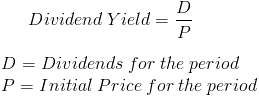# Dividend Yield (Stock)

Dividend Yield Calculator (Click Here or Scroll Down)The formula for the dividend yield is used to calculate the percentage return on a stock based solely on dividends. The total return on a stock is the combination of dividends and appreciation of a stock.

The dividends paid for a company can be found on the statement of retained earnings, which can then be used to calculate dividends per share.

## Use of Dividend Yield Formula

The dividend yield formula can be used by investors who are looking for increasing or declining trends of the dividend yield. On a broader level, a company that is paying less in dividends relative to its price may be having problems or it could be retaining more of a percentage of its net income for growth. When evaluating a stock, it is important to consider the overall company and how much net income it is retaining as reinvesting its net income could lead to growth and an appreciation of the stock price.

The formula for dividend yield may be of greater interest to investors who rely on dividends from their investments. However, a lower dividend yield does not imply lower dividends as the price could have substantially increased. As stated before, a trend of a declining dividend yield should only warrant investigation and not an immediate dismissal of the investment.

## Example of Dividend Yield Formula

An example of the dividend yield formula would be a stock that has paid total annual dividends per share of \$1.12. The original stock price for the year was \$28. If an individual investor wants to calculate their return on the stock based on dividends earned, he or she would divide \$1.12 by \$28. Using the formula for this example, the dividend yield would be 4%.

New to Finance?

Start with the Basics